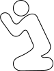CodePlea
Random thoughts on programming
23 Aug 2016

# Exponentiation Associativity and Standard Math Notation

Someone recently told me that TinyExpr (my math expression evaluation library) had several bugs, mostly related to the precedence and associativity of the exponentiation operator. It turned out that they thought that math notation was more standardized than it actually is.

I made TinyExpr to be used in a spreadsheet-like program. Therefore, I tried to make it somewhat compatible with spreadsheets. This makes for behavior that may surprise some people. For example, TinyExpr evaluates multiple exponentiation from left-to-right by default. This behaviour is clearly documented in the Readme and can be changed with a compile-time flag.

I thought it'd be nice to do a survey of common computer languages and tools and see how they handle exponentiation precedence and associativity.

## Left or Right Associativity of the Exponentiation Operator?

Left exponentiation associativity works like this: 2^2^3 == (2^2)^3 == 64.

Right exponentiation associativity works like this: 2^2^3 == 2^(2^3) == 256.

Many people would say that right associativity is more useful since (a^b)^c == a^(b*c) anyway. However, which is correct? I'd say neither is correct, because there is no widely agreed upon standard.

Let's see how other people handle it:

What Code Tested Result Associativity
Bash 2**2**3 256 right-associative
C++ a^b^c 64 left-associative
DuckDuckGo 2^2^3 256 right-associative
Excel 2^2^3 64 left-associative
EtherCalc 2^2^3 64 left-associative
Fortran 2**2**3 256 right-associative
Google 2^2^3 256 right-associative
Google Sheets 2^2^3 256 right-associative
Hand-held Calculators Varies
Lua 2^2^3 256 right-associative
Matlab 2^2^3 64 left-associative
Octave 2^2^3 64 left-associative
Perl 2**2**3 256 right-associative
PostgreSQL 2^2^3 64 left-associative
Python 2**2**3 256 right-associative
Ruby 2**2**3 256 right-associative
Tcl 2**2**3 256 right-associative
WolframAlpha 2^2^3 256 right-associative

Notes

• Am I being disingenuous in the C++ example? I don't think so. C++ programmers write this stuff.
• There is an interesting writeup on TCL here.

## Variable Negation or Exponentiation First?

Another apparent issue is what to do first in an expression such as -2^2. Should it be (-2)^2 == 4 or -(2^2) == -4? Let's find out!

What Code Tested Result First Step
Bash -2**2 4 negation
DuckDuckGo -2^2 4 negation
Excel -2^2 4 negation
EtherCalc -2^2 4 negation
Fortran -2**2 -4 exponentiation
Google -2^2 -4 exponentiation
Google Sheets -2^2 4 negation
Handheld Calculators -2^2 Varies
Lua -2^2 -4 exponentiation
Matlab -2^2 -4 exponentiation
Octave -2^2 -4 exponentiation
Perl -2**2 -4 exponentiation
PostgreSQL -2^2 4 negation
Python -2**2 -4 exponentiation
Ruby -2**2 -4 exponentiation
Tcl -2**2 4 negation
WolframAlpha -2^2 -4 exponentiation

## Conclusions

I don't think you can claim any one method is correct or incorrect. There simply is no widely agreed-upon standard.

For this reason I've made TinyExpr default to Excel-like behaviour, but it can be changed with a compile-time flag.

Also, you should really just use parentheses. If you don't, you're going to have a bad day sooner or later.

Like this post? Consider following me on Twitter or following me on Github. Don't forget to subscribe to my feed.